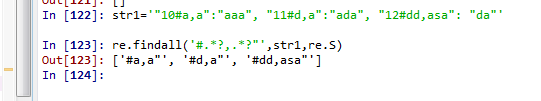###### Faiz2

2019-12-14 12:53 阅读 965

# 使用正则表达式匹配一段json字符串中是否有包含的字符

40

``````{"10#a,a":"aaa", "11#d,a":"ada", "12#dd,asa": "da"}
``````

``````/((?<=#).*?(?="))/g
``````

``````/((?<=#),(?="))/g
``````

=============分割线===============

``````/((?<=#).*?,.*?(?="))/g
``````
``````/((?<=#).*?\,.*?(?="))/g
``````
``````/((?<=#).*?[,].*?(?="))/g
``````
• 点赞
• 写回答
• 关注问题
• 收藏
• 复制链接分享

#### 1条回答默认 最新

• 本来.*？匹配的是a,a这三个字符，你改后只能匹配到#,"这样的东西了，#”之间只能有一个逗号很显然不存在这样的字符，所以失败。

/((?<=#).*？,.*？(?="))/g ，我不确定逗号要不要转义，你试下吧我在python里面试三个都能出来。（a,a 能出来。不管怎么样d,a 跟他一样的结构总该出来吧。）

补充：
Python里面括号表示要截取的内容，看你这个写的好像括号表示不截取。那你试试这样写
/((?<=#)(.*?),(.*?)(?="))/g

点赞 评论 复制链接分享